## The Lagrangian for Electromagnetic Fields

There are not many ways to make a scalar Lagrangian from the field tensor. We already know that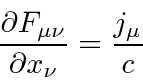and we need to make our Lagrangian out of the fields, not just the current. Again,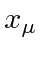cannot appear explicitly because that violates symmetries of nature. Also we want a linear equation and so higher powers of the field should not occur. A term of the formis a mass term and would cause fields to fall off faster than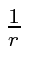. So, the only reasonable choice isOne might consider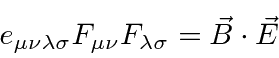but that is a pseudo-scalar, not a scalar. That is, it changes sign under a parity transformation. The EM interaction is known to conserve parity so this is not a real option. As with the scalar field, we need to add an interaction with a source term. Of course, we know electromagnetism well, so finding the right Lagrangian is not really guess work. The source of the field is the vector, so the simple scalar we can write is.

The Lagrangian for Classical Electricity and Magnetism we will try is.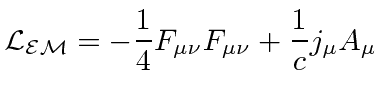In working with this Lagrangian, we will treat each component ofas an independent field.

The next step is to check what the Euler-Lagrange equation gives us.Note that, since we have four independent components of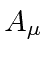as independent fields, we have four equations; or one 4-vector equation. The Euler-Lagrange equation gets us back Maxwell's equation with this choice of the Lagrangian. This clearly justifies the choice of.

It is important to emphasize that we have a Lagrangian based, formal classical field theory for electricity and magnetism which has the four components of the 4-vector potential as the independent fields.We could not treat each component ofas independent since they are clearly correlated. We could have tried using the six independent components of the antisymmetric tensor but it would not have given the right answer. Using the 4-vector potentials as the fields does give the right answer. Electricity and Magnetism is a theory of a 4-vector field.

We can also calculate the free field Hamiltonian density, that is, the Hamiltonian density in regions with no source term. We use the standard definition of the Hamiltonian in terms of the Lagrangian.We just calculated above thatwhich we can use to getWe will use this once we have written the radiation field in a convenient form. In the meantime, we can check what this gives us in general in a region with no sources.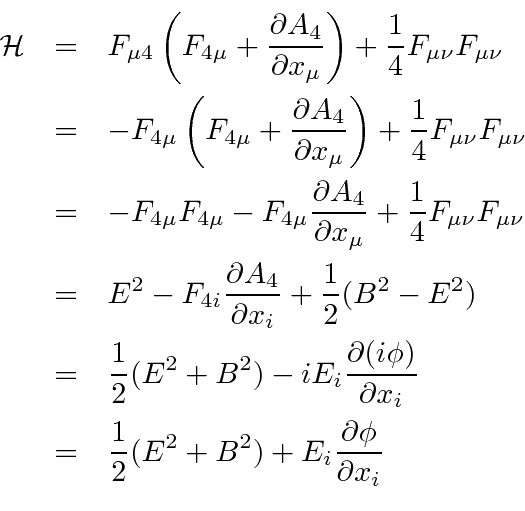If we integrate the last term by parts, (and the fields fall to zero at infinity), then that term contains awhich is zero with no sources in the region. We can therefore drop it and are left withThis is the result we expected, the energy density and an EM field. (Remember the fields have been decreased by a factor of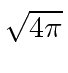compared to CGS units.)

We will study the interaction between electrons and the electromagnetic field with the Dirac equation. Until then, the Hamiltonian used for non-relativistic quantum mechanics will be sufficient. We have derived the Lorentz force law from that Hamiltonian.Jim Branson 2013-04-22